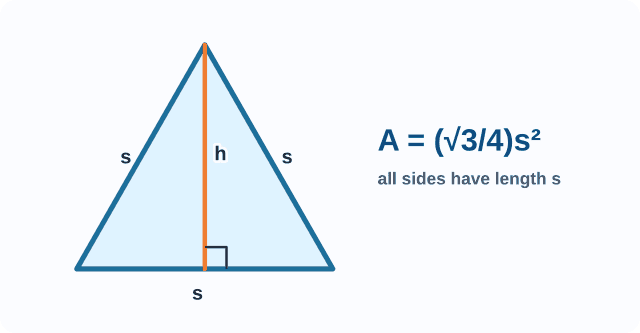index: click on a letter A B C D E F G H I J K L M N O P Q R S T U V W X Y Z A to Z index index: subject areas numbers & symbols sets, logic, proofs geometry algebra trigonometry advanced algebra & pre-calculus calculus advanced topics probability & statistics real world applications multimedia entrieswww.mathwords.com about mathwords website feedback

Equiangular Triangle

A triangle with three congruent angles.

Note: In Euclidean geometry, all equiangular triangles are equilateral and vice-versa. The angles of a Euclidean equiangular triangle each measure 60°.

 Equiangular (Equilateral) Triangle s = length of a side